## Written By: Lance Piatt```Excerpt from Rigging Lab Academy's Force Multipliers
Video is at the bottom```

I want to look at how we add vectors together. Previously, we discussed that if someone describes a displacement where they had two miles north and one mile east, we can add those together to get that endpoint. I want to look at that graphically now and give a few examples.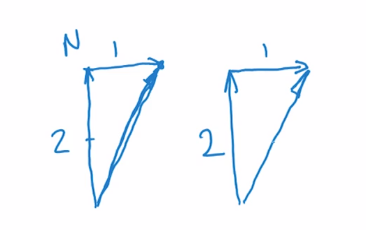Figure A

All we did when we did that, if someone moves two miles north, and I represent that by a line that might be two units long in a direction north, and then they go one mile to the east, so I’ve made that line there the same length as half of this one, then we know that their finished position is here.

And it can be described by that vector that we can draw there, that resultant vector. So we said that was two and that was one. What we did then is, effectively, I’ve taken two separate vectors, this one running two north. And then the second part of that description, I’ve got the tail of my new vector, the tail and the tip. I’ve got the tail of that vector and put it at the tip of the first one to draw in here, one. And then when I add those together, they come up with this final vector that describes that overall displacement.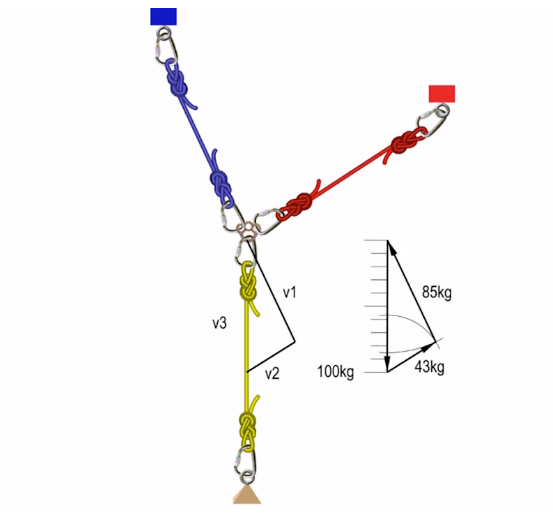Figure B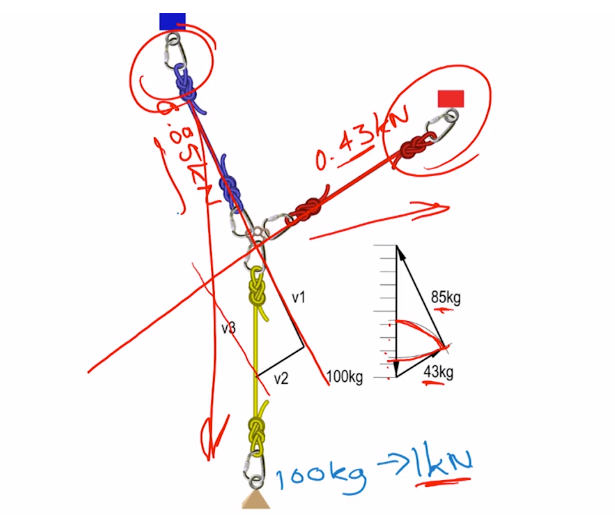Figure C

Now, I’ve got a couple of really relevant examples on the next slide (Figure B-C). This first one I’m going to start with, it’s pretty similar to the one we discussed a minute ago, where we’ve got two overhead anchors with an anchor focal point where this rigging plate is. And then we’ve got our load suspended below at the bottom of this yellow rope. So this is sort of the reverse vector edition. I want to work out if I hang a particular load here, and I’m going to say that’s a mass of 100 kilograms, and as we said before, that means we’ve got a weight of one kilonewton, if I hang that off that system, how much am I going to have in the tension in each of these strands of rope?

Well, I can solve this graphically if I take this line here, I might just change colors to make it a bit clearer, change to red, if I take this line and continue it in a straight line through my rigging plate. And I’ll do the same with my blue rope here. And then I’m going to turn that into a parallelogram. I want this line here, you’ll see that I’ve got this V1 already pre-drawn, the V2, sorry, the V1, I can now add in here as well. And the triangle that we’ve got drawn, the side lengths of this triangle actually define the ratio of the forces that apply to each anchor. And what I’ve depicted with this triangle is I’ve drawn a scale where that’s 10 arbitrary units long, it’s the same size as that triangle. And then I just want to measure the relative lengths of each of these sides.

So I’ve just drawn a radius into there and I can count up that scale. I’ve measured it, and it’s actually 43. And I’ll do the same with the other one. I can radius that one in and I can count down from the bottom to see that one is 85. So the relative side lengths of this triangle tell us exactly the ratio of the tensions in each anchor leg for the load that we’re applying in tension to our loaded strand. So for our one kilonewton weight that we’re suspending here, I can rewrite that and say that we would have 0.43 kilonewtons in this leg and 0.85 kilonewtons in this leg.Logically, that makes sense. If we imagine that system where everything is hanging, we can see that, well, this anchor should be taking most of the load because the load is almost suspended underneath it. And this one here, it’s effectively just acting as a redirection, pulling it sideways a little bit. So that, obviously, will bear less than what our blue anchor did.

A few more examples. This time, for each of these examples, I want to consider the position of this rigging plate between each of the people in each example. Is it going to be stationary or is it going to move?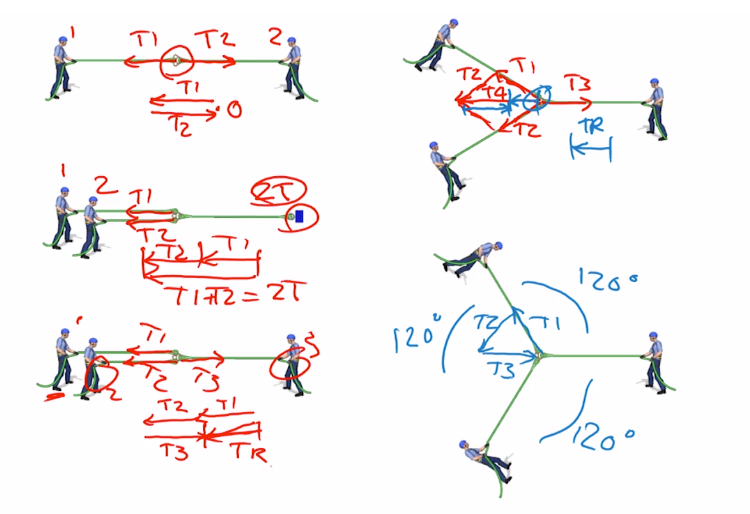Let’s assume (figure 1) that each person can pull with the same amount of tension. And it doesn’t matter what length I choose, as long as when I’d represent this vector, it’s going to be an arbitrary length, and let’s draw it that long there. So this person here, person two, I’m going to say is pulling with tension T2. And this person one, is pulling with tension T1 in this direction. When I add those two vectors together, remember we said I get the first vector and I draw that in place, and then the second vector, I’m going to put the tip of the first to the tail of the second. And I’ll put them there in there, T1 and T2. And this gets us back to the start. So the resultant is zero and that anchor plate isn’t going to move at all. I work through these other examples as well.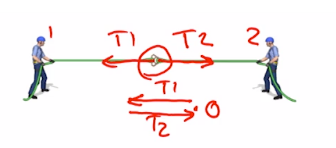Figure 1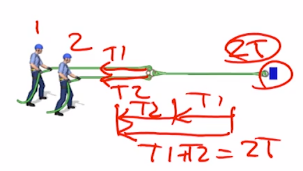Figure 2

This time (figure 2) we’ve got a fixed anchor and we’ve got two people, person one and person two. Person one’s applying T1, person two is applying T2. There’s T1. There’s T2. When I add those together, I draw T1 and then I’ll add T2 onto there. And the resultant that I get is actually that long, T1 plus T2. Let’s just call it 2T if each of those is pulling one unit of tension. So our anchor is going to feel two units of tension, which logically makes sense, but we’ve just demonstrated that by drawing the vectors and adding them.

What about this third one (figure 3), where we’ve got person one, person two, and person three? Person one is going to be pulling with T1, person two with T2, and person three with T3. I’ll add these three vectors together. T1 is there, T2 is there, and then T3 is coming back the other way. So the net result, if I start from the tail of my first vector and go to the tip of my third vector, we’ll call that TR for the T resultant. And logically, this makes sense as well because, effectively, we’ve got one person, this person will cancel out this person, so we’ve got one person applying all of the tension.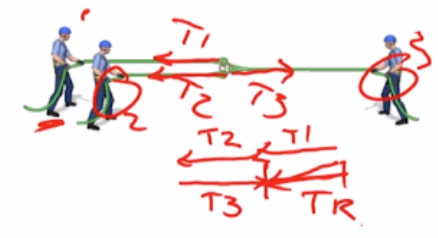Figure 3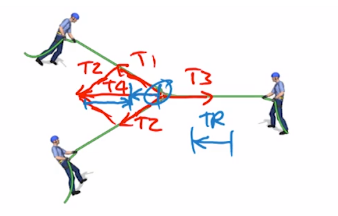Figure 4

Let’s move to our fourth example (figure 4). And again, I’ll just draw these in quickly. We’ll call that T3, T1, and T2. They’re all roughly the same length. I’m going to do this over the top of the ropes that I’ve got drawn. So I’m going to add T2 to T1. And it’s that same parallelogram situation that I had before. So that is parallel to that, and that is parallel to that. I’ll add T1 and T2 to get a resultant in here, which we’ll call T4. And now you can see if I just change colors, if I then superimpose T3, or add that to T4, so that’s at length of T3, I’m left with this vector in here. So our resultant going from the tail where we started to the tip of where we finished is going to be that vector, which I’ll just draw out here as our resultant vector.
So the rigging plate will move to the left, but it will experience less tension than if just one person was pulling on it, for example.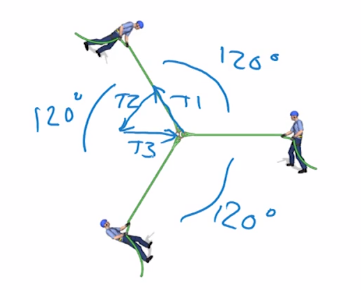Figure 5

The final example (figure 5), and looking at this, you should recognize that these angles here are about 120, which sort of rings bells for most of us as roping technicians. Everything should cancel out. Let’s check that by doing the vectors. So I’m going to have vector one coming up here, T1. Then I’m going to have my second one. So I’m going to draw that parallel to this T2 coming down here. Call that T2. And then this guy here is pulling that way with T3, which I can put in here, T3.So it finishes up back at the start again.

A couple of real-world photos from anchors that we set up at Smith Rock. I’m just going back to the red. I’ll just do these pretty quickly now that you’ve watched these examples. So I’m just going to pick an arbitrary length on this yellow rope and we’ll say we’ll go to there. And now I’m going to extend this rope through the anchor plate, and I’ll do the same with this one, extending through there. Now, this parallelogram, I want to construct so that it comes into this point here. So I’m going to draw one line there and one line there.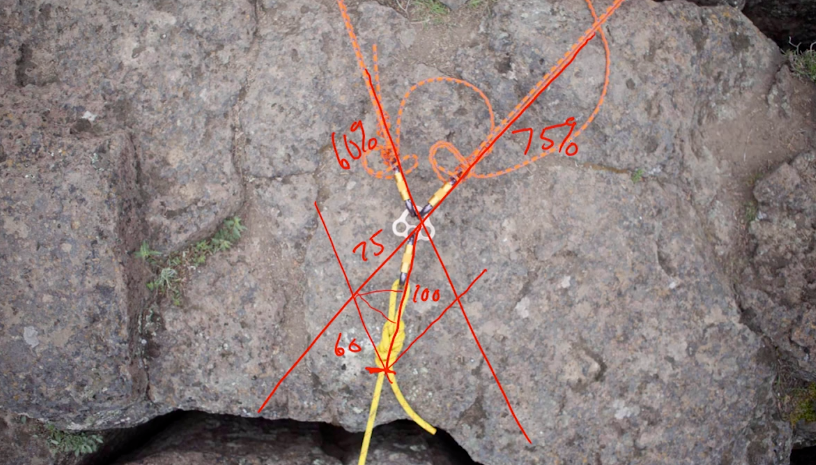Figure 6

So if I call this length here (figure 6), 100, and this is now where we can start to put in some estimates because as long as we’re within 10 or 20%, that’s good enough for what we’re doing in the field. Looking at these two here, I would say, and if I radius this around here, that looks like about 60. And if I take this one around here, that looks like about 75. So what does that mean? This anchor running back this way is going to take 60% of the load, and this one up here is going to take 75% of the load.

We’ve got three more examples like that. Logically, we should be thinking with this one that this anchor here is going to be taking most of that load because it’s almost in line and this one is just pulling a little bit to the side, but let’s do our checks. So I’ll extend this line through the anchor and I’ll extend this line through the anchor. I’ll choose my arbitrary length as 100% at the base of the knot. And I want to draw this line parallel to this one here. That’s pretty close. And the same thing with this one.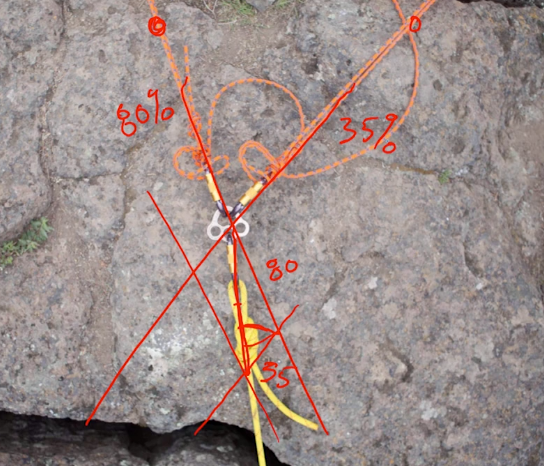Figure 7

So if this is 100 (figure 7), how long is that one there? If that was 50 there, then that looks like that’s about 35. And this one here looks like it’s about 80. So that means that this anchor, and logically, we sort of worked that out before, that takes about 80%, and this takes about 35%.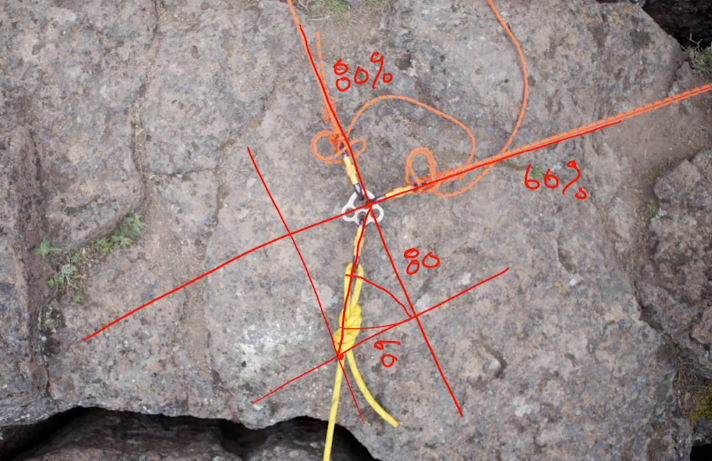Figure 8

Next one (figure 8), a little bit quicker this time, extend this one through the anchor focal point and this one as well. Our knot is our endpoint. Draw parallel to this line here. And the same thing parallel to this line. I’ll just analyze one side of this triangle. I’ll radius this one in. That looks like about 60, and this one looks like about 80.

So 80% on this anchor and 60% on this anchor. That one might be anywhere between 65 and 90 and this one might be between 40 and 70. That’s near enough. The last one that we’ll do should be setting off some warning bells for that really large angle between these two, but let’s see what it tells us. So running through the anchor here and here. My arbitrary 100, I’m going to put at the base of the knot there. It could be anywhere. It could be out here or it could be in here, but let’s go there.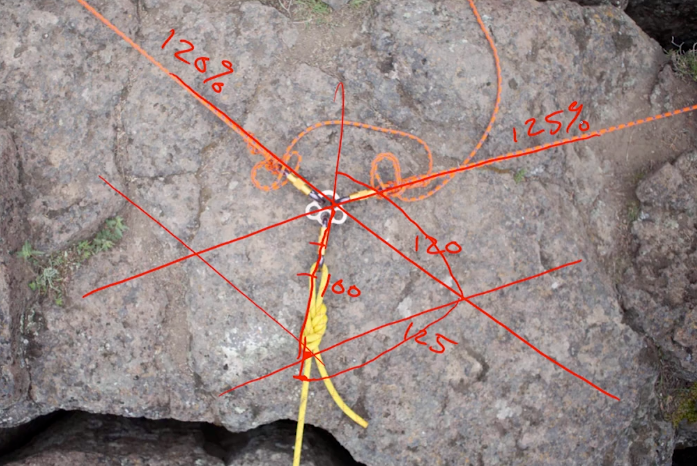Figure 9

I’ll try and get these lines parallel. And this guy here. Okay. So now we can see that if that’s 100 in the middle, when I radius this around, it looks like it’s about 125. And then the same with this one. Bring that into the line of the yellow rope. So from here to here, that looks like about 120. So that tells us that this anchor here is taking 120% of the applied load and this one here is taking 125% of the applied load.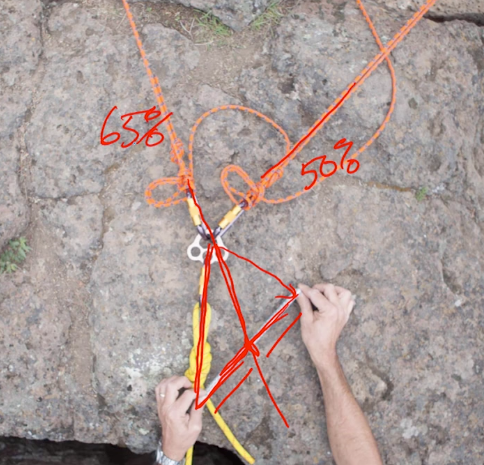Figure 10

So this is all well and good if we can get above and take a photo, and use some really fancy apps that you can get like RigRite (it’s a really cool app for working your stuff out in the field if you’ve got a smartphone and can take a photo). But look, to be honest, we’re often in places where we don’t want to rely on the technology to do that. So here’s a quick and dirty way that we can do it in the field (figure 10).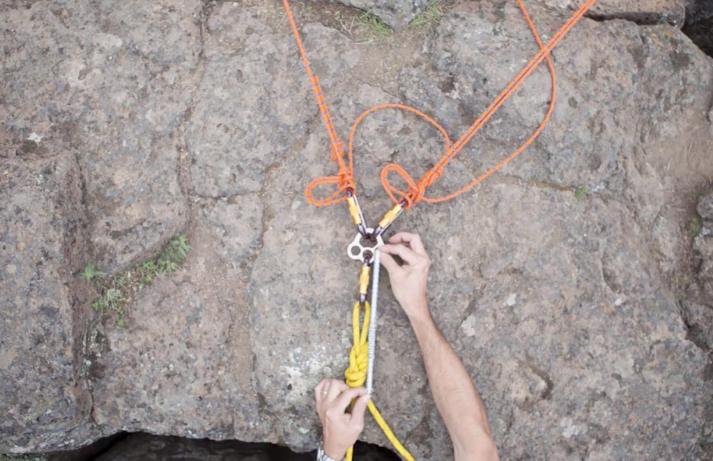Figure 11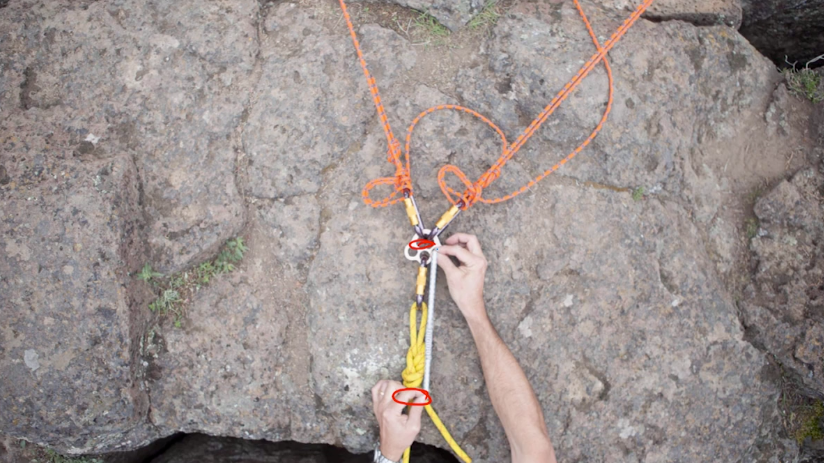Figure 12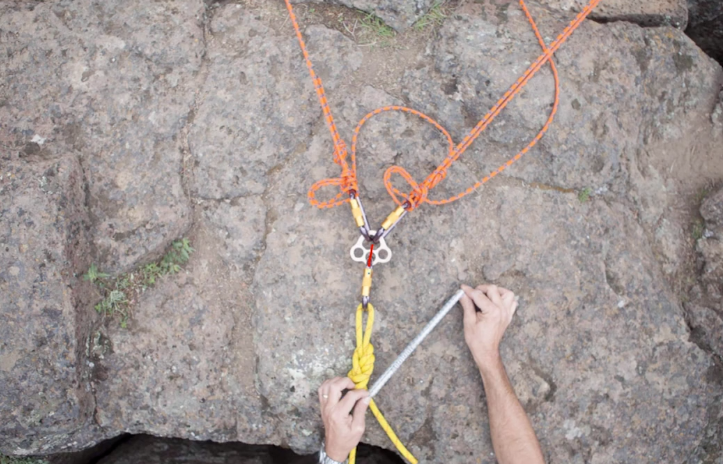Figure 13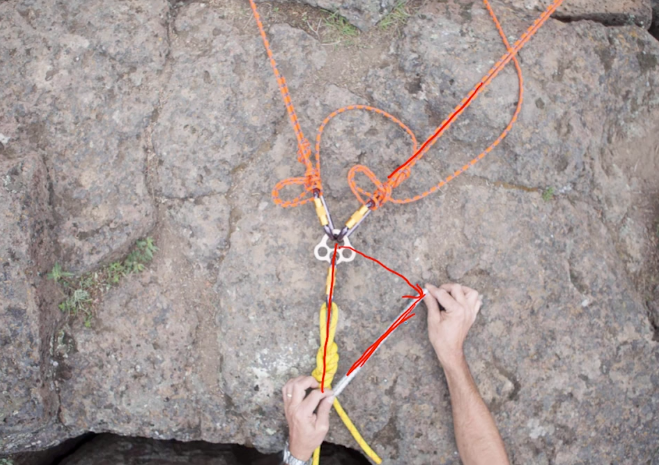Figure 14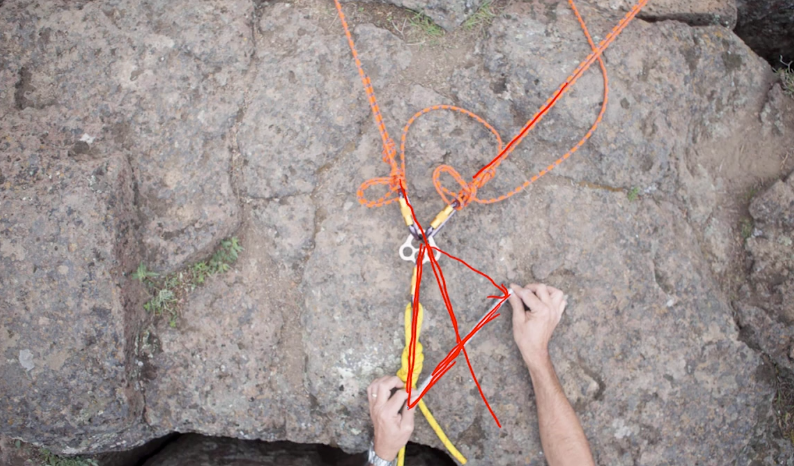Figure 15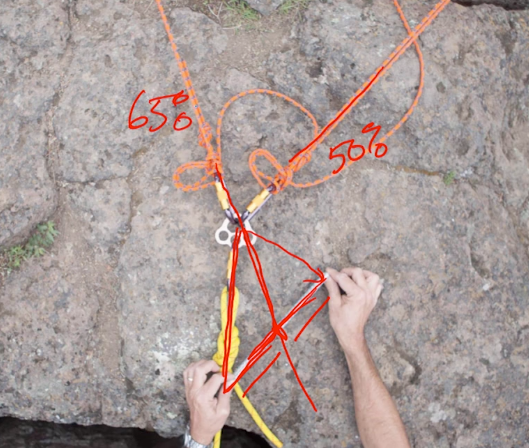Figure 16

So all I’ve got is a short sling (Figure 11-12) that I’ve started from the base of the knot to the anchor focal point there.

And then in the next slide, you’ll just see that I’ve spun that sling around (Figure 13).

So I’ve got my reference length of 100, and I’ve moved that around on a radius until that is parallel with one of our anchor legs. Okay. So now that lets me visualize the situation where I can draw a line in the dirt, or I can put a stick there and continue this one through to where it hits my sling.

And now I’ve got my three sides of my triangle (Figure 14-15). And I can see straight away that has hit about halfway along the length of that, which means that this anchor here is going to take 50% of the load. And while I’m just holding my sling there, I can see similarly that this one, the longer one, looks like it’s about 65% (Figure 16). So that’s going to take 65% of the load.

So whether it’s using a sling or a pen or a twig that you can break when you pick it up off the ground or just draw the lines in the dirt underneath the anchor, it’s a really speedy way to work out what your relative load distribution on your anchor points is with a particular applied loan.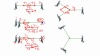Lance

##### Categories

1.